The Rocscience International Conference 2021 Proceedings are now available. Read Now

Batch Compute

This tutorial will familiarize you with the Batch Compute feature of UnWedge by Batch Computing from select UnWedge files.

Topics Covered in this Tutorial:

• Adding Input UnWedge Model(s) to the Batch Compute File Queue
• Output UnWedge Batch Compute Results File

Finished Product:

The finished products of this tutorial can be found in the Tutorial 12 Batch Compute folder, located in the Examples > Tutorials folder in your UnWedge installation folder. Inside, you will find:

• Tutorial 01 Quick Start.weg5 UnWedge model file
• Tutorial 04 Joint Combinations.weg5 UnWedge model file
• Tutorial 07 Probabilistic Analysis.weg5 UnWedge model file
• Tutorial 01 Quick Start folder containing the computed results files
• Tutorial 04 Joint Combinations folder containing the computed results files
• Tutorial 07 Probabilistic Analysis folder containing the computed results files

1.0 Introduction

The Batch Compute option helps users quickly generate results for several UnWedge model files at a time.

2.0 Batch Compute

If you have not already done so, run the UnWedge program by double-clicking the UnWedge icon in your installation folder or by selecting Programs > Rocscience > UnWedge > UnWedge in the Windows Start menu.

2.1 UNWEDGE FILES

For the purpose of demonstration, we will run Batch Compute on the three UnWedge tutorial files that were already created for you, located in the Examples > Tutorials folder in your UnWedge installation folder:

• Tutorial 01 Quick Start.weg5
• Tutorial 04 Joint Combinations.weg5
• Tutorial 07 Probabilistic Analysis.weg5

Let's create a folder called Tutorial 12 Batch Compute and copy the three UnWedge tutorial files to this folder.

2.2 ADD FILES TO FILE QUEUE

The Batch Compute dialog allows you to add UnWedge model files to the queue, select a an output format, and compute the results.

To add multiple files to the File Queue:

1. Select Batch Compute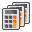from the File menu to open the Batch Compute dialog.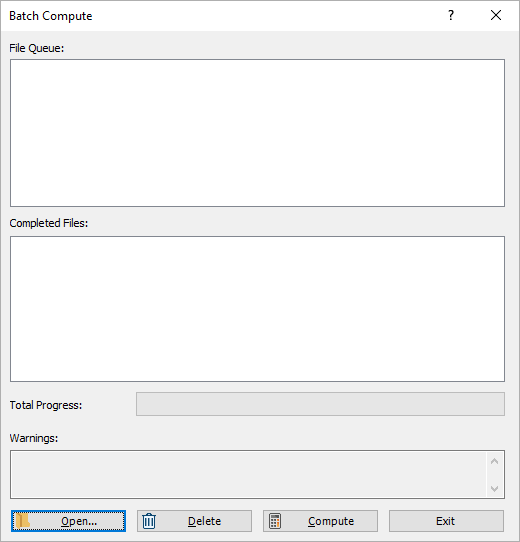2. Select the Open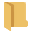button to open a standard dialog to choose a directory. Navigate to the Tutorial 12 Batch Compute folder containing the three UnWedge tutorial files and Select Folder .

You will see the three UnWedge files appear under the File Queue list box.3.0 Output Result Files

Results files are automatically generated and saved into the same directory as the input model files after Batch Compute is complete. The output files are in Excel format.

3.1 EXCEL FILE FORMAT

While still in the Batch Compute dialog:

1. Select the Compute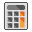button to start the batch computing process.
2. The Total Progress can be seen at the bottom of the dialog. When files have finished computing, they will appear in the Completed Files list box.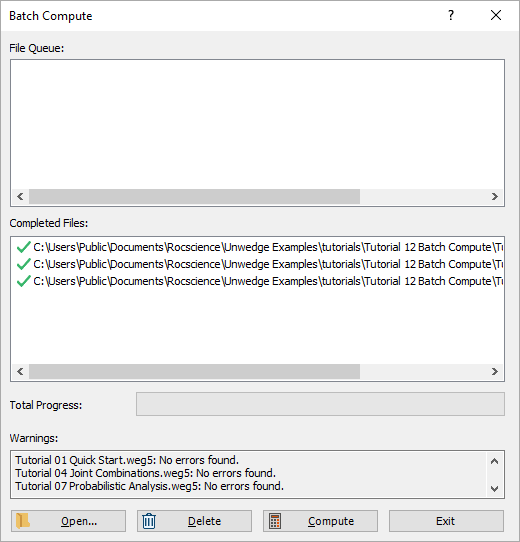3. When all files have finished computing, select Exit to close the dialog.

NOTE: Probabilistic models may take a few minutes to compute.

For each input UnWedge model file, an Excel file (.xlsx) containing all results data is generated.

• Take a look at the Results.xlsx result files.

For a Deterministic Analysis with only 1 possible joint combination ( Tutorial 01 Quick Start.weg5), only 1 row of results data is outputted following the header row for each possible wedge. Each data type is separated by column.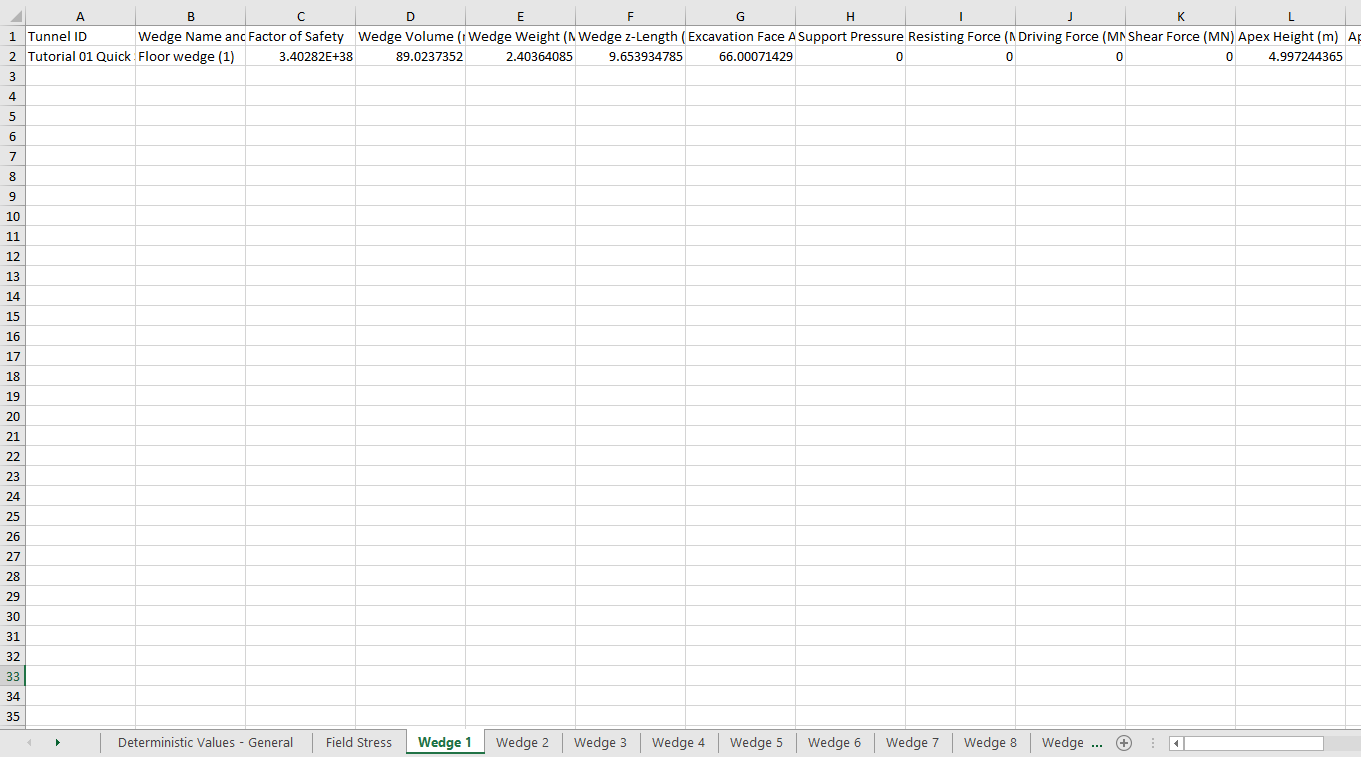For a Deterministic Analysis with multiple joint combinations ( Tutorial 04 Joint Combinations.weg5), all joint combinations are outputted following the header row (in each of the wedge sheets). Each row of results data corresponds to a joint combination. Each data type is separated by column. In this model, all 10 possible joint combinations results are contained within the output file.For a Probabilistic Analysis ( Tutorial 07 Probabilistic Analysis.weg5), all probabilistic samples are outputted following the header row (in each of the wedge sheets). Each row of results data corresponds to a sample wedge. Each data type is separated by column. In this model, all 10,000 sample results are contained within the output file.This concludes the tutorial. You are now ready for the next tutorial, Tutorial 13 - Automate Deterministic Analysis from Excel in UnWedge.